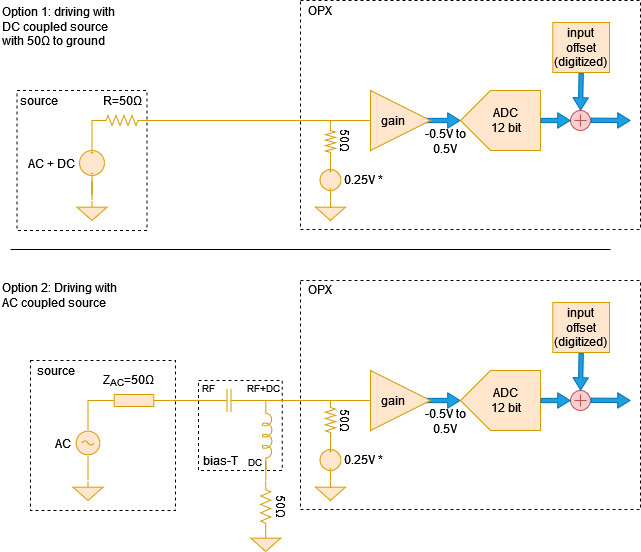The signals acquired by the OPX using the analog input channels are first passed through an analog input stage and then digitized. Here we discuss the voltage limits, gain and offset calibrations that can be applied to the signal to be digitized.

## Acquiring analog signals with the OPX¶The signal chain can be seen in Fig. 1. The signal source can be either AC or DC coupled.

Note

The OPX input has a common-mode voltage of 0.25V when measured with 50ohm.

• DC coupled signal source

If the signal source is DC coupled and has 50ohm DC resistance to ground, it can be directly connected to the analog input. This can be seen in Option 1 of Fig. 1

• AC coupled signal source

If the signal source is AC coupled and does not have a 50ohm resistance to ground, a bias T is needed in order to bias the input amplifier. The required setup can be seen in option 2 of Fig. 1. In this case, the source will still need to have a 50ohm AC impedance throughout its bandwidth for proper operation.

• Input impedance: 50ohms
• Input bandwidth: 400MHz
• Full voltage range: 1.0Vpp into 50ohm or 4dBm (nominal)
• Variable gain: -12dB to 20dB in steps of 1dB

## Setting the voltage gain¶

The voltage gain can be set by adding a "gain_db" field to the "analog_inputs" field of the controller in the config. Here we show an example with the relevant fields:

config = {
'version': 1,
'controllers': {
'con1': {
'analog_inputs': {
1: {'offset': 0.0, 'gain_db': -1},
2: {'offset': 0.0, 'gain_db': 1},
}
}
},
}


Note

When changing the gain, the input DC offset needs to be recalibrated.